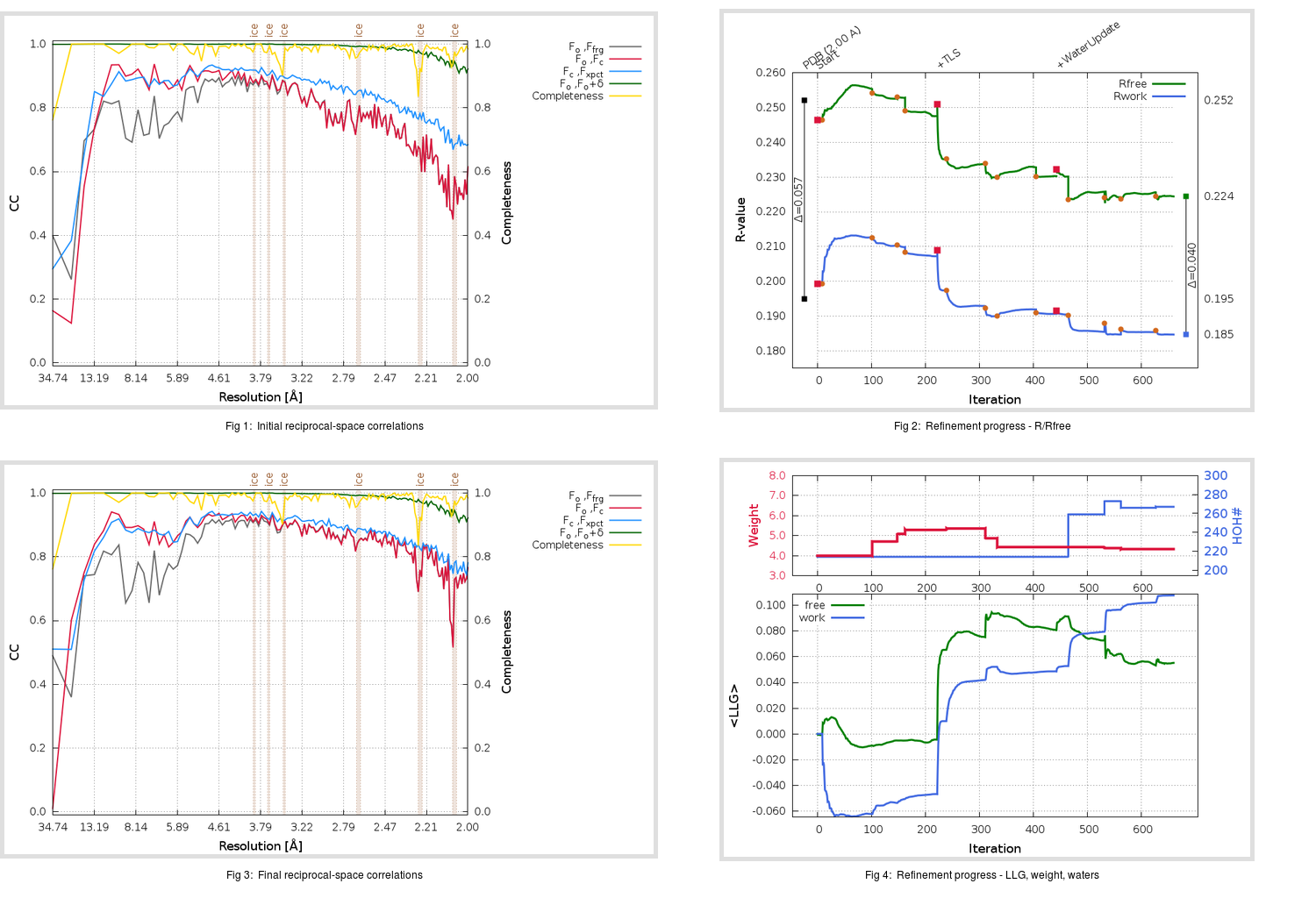Content:

## Deposited

` `
 Date deposited Date data collection Resolution R, Rfree 20170214 20150525 2.00 0.1920 0.2520

Molprobity (CCP4 7.0 version) summary:

```Ramachandran outliers =   0.00 %
favored =  96.38 %
Rotamer outliers      =   5.30 %
C-beta deviations     =     3
Clashscore            =   0.63
RMS(bonds)            =   0.0199
RMS(angles)           =   1.91
MolProbity score      =   1.50
Resolution            =   2.00
R-work                =   0.1920
R-free                =   0.2520
```

```Number of waters      =   214

<B> (all atoms) =   45.45 ( sd =   12.82 ) for       2630 non-hydrogen atoms
<B>   (protein) =   44.71 ( sd =   12.73 ) for       2377 non-hydrogen atoms
<B>     (water) =   52.36 ( sd =    9.28 ) for        214 non-hydrogen atoms
<B>    (others) =   52.11 ( sd =   19.85 ) for         39 non-hydrogen atoms

B min/max       (all non-hydrogen atoms) =   24.37 /  114.82
B min/max   (protein non-hydrogen atoms) =   24.37 /  114.82
B min/max     (water non-hydrogen atoms) =   31.14 /   72.87
B min/max     (other non-hydrogen atoms) =   35.55 /   97.43
```

## BUSTER (re-)refinement

` `

Molprobity (CCP4 7.0 version) summary:

```Ramachandran outliers =   0.00 %
favored =  97.37 %
Rotamer outliers      =   4.17 %
C-beta deviations     =     1
Clashscore            =   1.48
RMS(bonds)            =   0.0111
RMS(angles)           =   1.58
MolProbity score      =   1.48
Resolution            =   2.00
R-work                =   0.1847
R-free                =   0.2244
```

```Number of waters      =   267

<B> (all atoms) =   44.31 ( sd =   12.23 ) for       2683 non-hydrogen atoms
<B>   (protein) =   42.91 ( sd =   11.30 ) for       2377 non-hydrogen atoms
<B>     (water) =   56.98 ( sd =   13.51 ) for        267 non-hydrogen atoms
<B>    (others) =   43.08 ( sd =    6.27 ) for         39 non-hydrogen atoms

B min/max       (all non-hydrogen atoms) =   23.98 /  132.95
B min/max   (protein non-hydrogen atoms) =   23.98 /  132.95
B min/max     (water non-hydrogen atoms) =   26.37 /  112.80
B min/max     (other non-hydrogen atoms) =   35.45 /   55.85
```

Refinement progression:Results:

` `
 File Remark 5N5O_aB_refine.01_03_refine.pdb.gz exact refinement commands are in header 5N5O_aB_refine.01_03_refine.mtz.gz including original deposited data and several re-refinement map coefficients 5N5O_aB_refine.01_03_BUSTER_model.cif.gz including any non-standard compound restraints 5N5O_aB_refine.01_03_BUSTER_refln.cif.gz